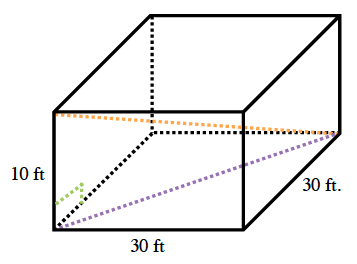### Home > CC3MN > Chapter 9 > Lesson 9.1.6 > Problem9-94

9-94.

Additional Challenge: Use the diagram and your answer to problem 9-88 above. If your classroom measured $30$ ft by $30$ ft by $10$ ft, what distance would the fly travel?Find the distance the fly traveled by finding the hypotenuse of the triangle (in orange).
Notice that the longest leg of the right triangle (in purple) is the distance between two opposite corners on the floor.

$\sqrt{1900} \approx 43.59\text{ ft.}$